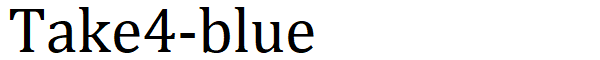# Creating a Garmin watch face: performance 4

Tips for improving performance.

After that, I also checked the forum to see if the above measurement was correct.
The forum stated that `System.getTimer()` would be used, but even when I actually tried it on the simulator, the processing time was 0 ms, and I could not determine whether it was correct or incorrect.

## Techniques for improving performance.

### Reduce the number of operations.

When performing the same operation more than once, it is better to assign the operation to a variable once and use it.
Looking at the performance, up to two times, if the same calculation was performed three or more times by using the value assigned to the variable, the performance improved by assigning to the variable.

#### Ordinary pattern

First, Creating a Garmin watch face: performance 3, but the following coding.

``````for (var i = 1; i < 180; i++) {
var now = [center + radius * Math.cos(baseAngle * i), center - radius * Math.sin(baseAngle * i)];
dc.drawLine(prev, prev, now, now);
prev = now;
}
``````

The angle given to sin / cos is performed each time each function is called.
This is common code, but in such a case, it is better to assign it to a variable once and then pass it to sin / cos.

``````for (var i = 1; i < 180; i++) {
var angle = baseAngle * i;
var now = [center + radius * Math.cos(angle), center - radius * Math.sin(angle)];
dc.drawLine(prev, prev, now, now);
prev = now;
}
``````

#### Pattern used for judgment in for statement

The same thing is often done about the comparison part of the for statement.
If an implementation that performs an operation is performed in this part, the performance will be reduced.

``````a = 5;
b = 4;
for (var i = 1; i < a * b; i++) {
System.println(i);
}
``````

In this case, since the operation of `a * b` is performed every time at the part of `i <a * b`, the performance is reduced.
Assign `a * b` to a variable before the for statement, and compare it with it.

Some functions are inconsistent with degrees and radians.
I think it is better to do as follows.

``````var toRad = Math.PI / 180.0;
``````

A method of preparing constants for degree-radian conversion in toRad and calculating using them.
Math had a conversion function, but the above implementation was about 10ns faster.

### Drawing process

#### Drawing text

Drawing text took longer as the font size was larger.
The processing time was the same, but the graphics time was different.

#### Drawing an arc

This is because, as shown in Creating a Garmin watch face: performance 3, the overall processing time was faster when drawing and decomposing into line segments using drawLine instead of drawArc.

## Finally

The performance enhancements listed here are based on the assumption that Monkey C has no compiler optimizations. It could not be determined whether the optimization could be performed.

If optimizations become available in the future, such implementation techniques may not be needed.